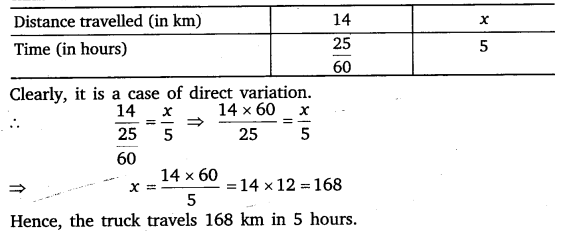# Class 8 Maths NCERT Solutions for Chapter – 13 Direct and Inverse Proportions Ex – 13.1

## Direct and Inverse Proportions

Question 1.
Following are the car parking charges near a railway station upto
4 hours          ₹ 60
8 hours          ₹ 100
12 hours        ₹ 140
24 hours        ₹ 180
Check if the parking charges are in direct proportion to the parking time.

Solution:∴ The parking charges are not in direct proportion to the parking time.

Question 2.
A mixture of paint is prepared by mixing 1 part of red pigments with 8 parts of base. In the following table, find the parts of base that need to be added.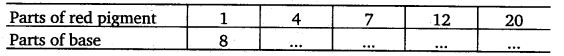Solution:
It is given that parts of red pigment, say x and parts of base, say y are in direct proportion. Therefore, the ratio of the corresponding values of x and y remain constant.
We have,$\frac { 1 }{ 8 } =\frac { 1 }{ 8 }$.
So, x and y are in direct variation with the constant of variation equal to$\frac { 1 }{ 8 }$. This means that x is$\frac { 1 }{ 8 }$ of y and y is eight times of x. Thus, the required entries are$\frac { 4 }{ 32 } ,\frac { 7 }{ 56 } ,\frac { 12 }{ 96 } ,\frac { 20 }{ 160 }$.
Thus, table becomesQuestion 3.
In Question 2 above, if 1 part of a red pigment requires 75 ml. of base, how much red pigment should we mix with 1800 ml of base?

Solution:
Suppose x parts of red pigment should be mixed with 1800 mL of base. Then the above information can be put in the tabular form as follows:Question 4.
A machine in a soft drink factory fills 840 bottles in six hours. How many bottles will it fill in five hours?

Solution:
Suppose x bottles be filled up in five hours. Then the information can be put in the following tabular form :We observe that the lesser the time consumed, the lesser the number of botdes filled up. So, it is a case of direct variation.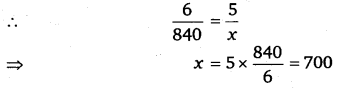Hence, 700 botdes will be filled up in 5 hours.

Question 5.
A photograph of a bacteria enlarged 50,000 times attains a length of 5 Cm as shown in the diagram. What is the actual length of the bacteria? If the photograph is enlarged 20,000 times only, what would be its enlarged length?

Solution: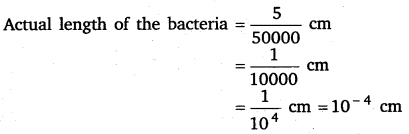Suppose x be the enlarged length of the bacteria when its photograph is enlarged 20000 times. Then the information can be put in the following tabular form :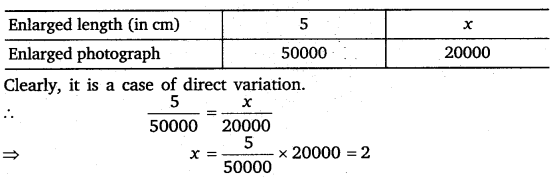Hence, its enlarged length is 2 cm.

Question 6.
In a model of a ship, the mast is 9 cm high, while the mast of the actual ship is 12 m high. If the length of the ship is 28 m, how long is the model ship?

Solution:
Let x metres be the length of the model ship of actual length 28 m. Then the information can be put in the following tabular form :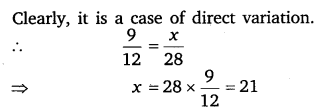Hence, the length of the model ship is 21 cm.

Question 7.
Suppose 2 kg of sugar contains 9 x 106 crystals. How many sugar crystals are there in
(i)
5 kg of sugar?
(ii) 1.2 kg of sugar?

Solution:
Let x and y crystals are in 5 kg of sugar and 1.2 kg of sugar. Then, the given information can be exhibited in the following tabular form :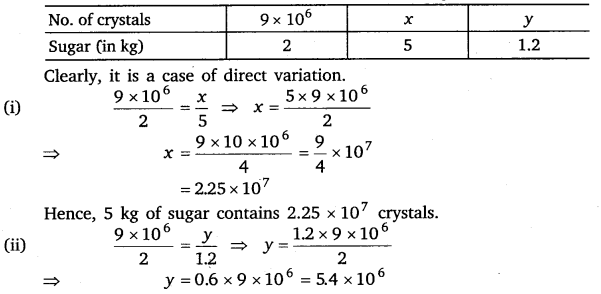Hence, 1.2 kg of sugar contains 5.4 x 106 crystals.

Question 8.
Rashmi has a road map with a scale of 1 cm representing 18 km. She drives on a road for 72 km. What would be her distance covered in the map?

Solution:
Let x cm be the distance covered in the map for the drives of 72 km on a road. Then, the given information can be exhibited in the following tabular form.Question 9.
A 5 m 60 cm high vertical pole casts a shadow 3 m 20 cm long. Find at the same time (i) the length of the shadow cast by another pole 10 m 50 cm high (ii) the height of a pole which casts a shadow 5 m long.

Solution:
Let x m be the length of the pole whose shadow is of length 10 m 50 cm.
Let y m be the length of the pole whose shadow is 5 m long.
Then, the given information can be exhibited in the following tabular form :Question 10.
A loaded truck travels 14 km in 25 minutes. If the speed remains the same, how far can it travel in 5 hours?

Solution:
Let the truck travels x km in 5 hours. Then, the given information can be exhibited in the following tabular form :